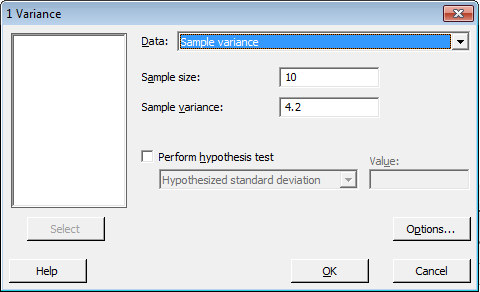# 4.1 - One Variance

4.1 - One Variance

Let's start right out by stating the confidence interval for one population variance.

Theorem

If $$X_{1}, X_{2}, \dots , X_{n}$$ are normally distributed and $$a=\chi^2_{1-\alpha/2,n-1}$$ and $$b=\chi^2_{\alpha/2,n-1}$$, then a $$(1−\alpha)\%$$ confidence interval for the population variance $$\sigma^2$$ is:

$$\left(\dfrac{(n-1)s^2}{b} \leq \sigma^2 \leq \dfrac{(n-1)s^2}{a}\right)$$

And a $$(1−\alpha)\%$$ confidence interval for the population standard deviation $$\sigma$$ is:

$$\left(\dfrac{\sqrt{(n-1)}}{\sqrt{b}}s \leq \sigma \leq \dfrac{\sqrt{(n-1)}}{\sqrt{a}}s\right)$$

### Proof

We learned previously that if $$X_{1}, X_{2}, \dots , X_{n}$$ are normally distributed with mean $$\mu$$ and population variance $$\sigma^2$$, then:

$$\dfrac{(n-1)S^2}{\sigma^2} \sim \chi^2_{n-1}$$

Then, using the following picture as a guide:

with ($$a=\chi^2_{1-\alpha/2}$$) and ($$b=\chi^2_{\alpha/2}$$), we can write the following probability statement:

$$P\left[a\leq \dfrac{(n-1)S^2}{\sigma^2} \leq b\right]=1-\alpha$$

Now, as always it's just a matter of manipulating the quantity in the parentheses. That is:

$$a\leq \dfrac{(n-1)S^2}{\sigma^2} \leq b$$

Taking the reciprocal of all three terms, and thereby changing the direction of the inequalities, we get:

$$\dfrac{1}{a}\geq \dfrac{\sigma^2}{(n-1)S^2} \geq \dfrac{1}{b}$$

Now, multiplying through by $$(n−1)S^2$$, and rearranging the direction of the inequalities, we get the confidence interval for $$\sigma ^2$$:

$$\dfrac{(n-1)S^2}{b} \leq \sigma^2 \leq \dfrac{(n-1)S^2}{a}$$

as was to be proved. And, taking the square root, we get the confidence interval for $$\sigma$$:

$$\dfrac{\sqrt{(n-1)S^2}}{\sqrt{b}} \leq \sigma \leq \dfrac{\sqrt{(n-1)S^2}}{\sqrt{a}}$$

as was to be proved.

## Example 32-1A large candy manufacturer produces, packages and sells packs of candy targeted to weigh 52 grams. A quality control manager working for the company was concerned that the variation in the actual weights of the targeted 52-gram packs was larger than acceptable. That is, he was concerned that some packs weighed significantly less than 52-grams and some weighed significantly more than 52 grams. In an attempt to estimate $$\sigma$$, the standard deviation of the weights of all of the 52-gram packs the manufacturer makes, he took a random sample of n = 10 packs off of the factory line. The random sample yielded a sample variance of 4.2 grams. Use the random sample to derive a 95% confidence interval for $$\sigma$$.

First, we need to determine the two chi-square values with (n−1) = 9 degrees of freedom. Using the table in the back of the textbook, we see that they are:

$$a=\chi^2_{1-\alpha/2,n-1}=\chi^2_{0.975,9}=2.7$$ and $$b=\chi^2_{\alpha/2,n-1}=\chi^2_{0.025,9}=19.02$$

Now, it's just a matter of substituting in what we know into the formula for the confidence interval for the population variance. Doing so, we get:

$$\left(\dfrac{9(4.2)}{19.02} \leq \sigma^2 \leq \dfrac{9(4.2)}{2.7}\right)$$

Simplifying, we get:

$$(1.99\leq \sigma^2 \leq 14.0)$$

We can be 95% confident that the variance of the weights of all of the packs of candy coming off of the factory line is between 1.99 and 14.0 grams-squared. Taking the square root of the confidence limits, we get the 95% confidence interval for the population standard deviation $$\sigma$$:

$$(1.41\leq \sigma \leq 3.74)$$

That is, we can be 95% confident that the standard deviation of the weights of all of the packs of candy coming off of the factory line is between 1.41 and 3.74 grams.

## Using Minitab

#### Confidence Interval for One Variance

1. Under the Stat menu, select Basic Statistics, and then select 1 Variance...:

2. In the pop-up window that appears, in the box labeled Data, select Sample variance. Then, fill in the boxes labeled Sample size and Sample variance.3. Click on the button labeled Options... In the pop-up window that appears, specify the confidence level and "not equal" for the alternative.4. Then, upon clicking OK on the main pop-up window, the output should appear in the Session window:

##### Test and CI for One Variance

Method

The chi-square method is only for the normal distribution.
The Bonett method cannot be calculated with summarized data.

Statistics

N StDev Variance
10 2.05 4.20

95% Confidence Intervals

Method CI for
StDev
CI for
Variance
Chi-Square (1.41,  3.74) (1.99,  14.00)

  Link ↥ Has Tooltip/Popover Toggleable Visibility